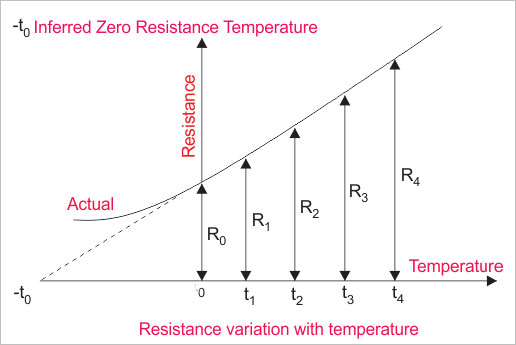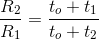# Resistance Variation with Temperature

There are some materials mainly metals, such as silver, copper, aluminum, which have plenty of free electrons. Hence this type of materials can conduct current easily that means they are least resistive. But the resistivity of these materials is highly dependable upon their temperature. Generally metals offer more electrical resistance if temperature is increased. On the other hand the resistance offered by a non-metallic substance normally decreases with increase of temperature.If we take a piece of pure metal and make its temperature 0o by means of ice and then increase its temperature from gradually from 0oC to to 100oC by heating it.

During increasing of temperature if we take its resistance at a regular interval, we will find that electrical resistance of the metal piece is gradually increased with increase in temperature. If we plot the resistance variation with temperature i.e. resistance Vs temperature graph, we will get a straight line as shown in the figure below. If this straight line is extended behind the resistance axis, it will cut the temperature axis at some temperature, – t0oC. From the graph it is clear that, at this temperature the electrical resistance of the metal becomes zero. This temperature is referred as inferred zero resistance temperature.
Although zero resistance of any substance cannot be possible practically. Actually rate of resistance variation with temperature is not constant throughout all range of temperature. Actual graph is also shown in the figure below.
Let’s R1 and R2 are the measured resistances at temperature t1oC and t2oC respectively. Then we can write the equation below,From the above equation we can calculate resistance of any material at different temperature. Suppose we have measured resistance of a metal at t1oC and this is R1.
If we know the inferred zero resistance temperature i.e. t0 of that particular metal, then we can easily calculate any unknown resistance R2 at any temperature t2oC from the above equation.

The resistance variation with temperature is often used for determining temperature variation of any electrical machine. For example, in temperature rise test of transformer, for determining winding temperature rise, the above equation is applied. This is impossible to access winding inside the an electrical power transformer insulation system for measurement of temperature but we are lucky enough that we have resistance variation with temperature graph in our hand. After measuring electrical resistance of the winding both at the beginning and end of the test run of the transformer, we can easily determine the temperature rise in the transformer winding during test run.

20oC is adopted as standard reference temperature for mentioning resistance. That means if we say resistance of any substance is 20Ω that means this resistance is measured at the temperature of 20oC.

### Video Resistance Variation with Temperature

Want To Learn Faster? 🎓
Get electrical articles delivered to your inbox every week.
No credit card required—it’s 100% free.